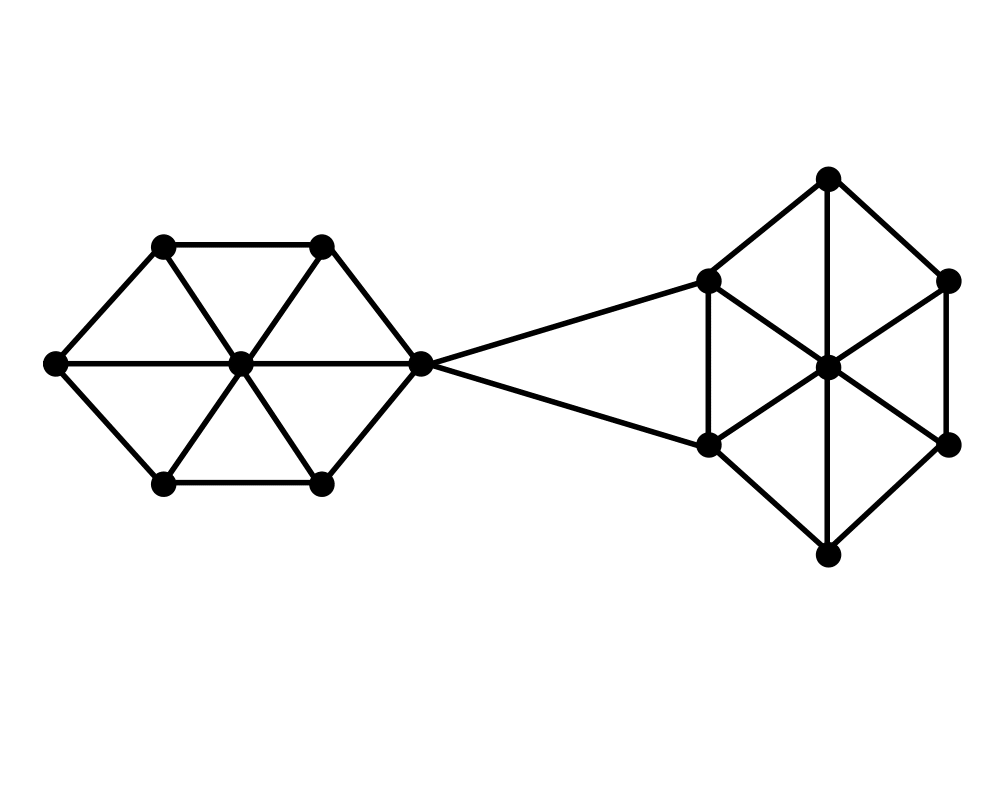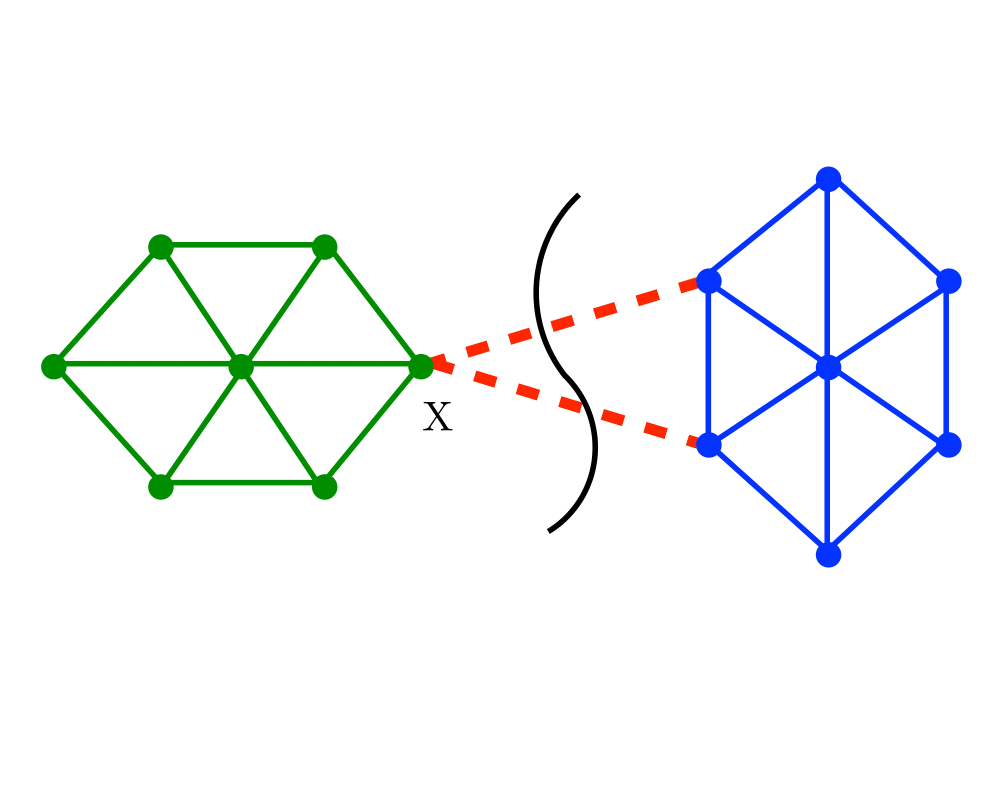# Edge and Vertex ConnectivityInputOutput

Input Description: A graph $$G$$. Optionally, a pair of vertices $$s$$ and $$t$$.
Problem: What is the smallest subset of vertices (edges) whose deletion will disconnect $$G$$? Alternately, what is the smallest subset of vertices (edges) which will separate $$s$$ from $$t$$?

Excerpt from The Algorithm Design Manual: Graph connectivity often arises in problems related to network reliability. In the context of telephone networks, the vertex connectivity is the smallest number of switching stations that a terrorist must bomb in order to separate the network, \ie prevent two unbombed stations from talking to each other. The edge connectivity is the smallest number of wires that need to be cut to accomplish the same thing. One well-placed bomb or snipping the right pair of cables suffices to disconnect the network above.

The edge (vertex) connectivity of a graph $$G$$ is the smallest number of edge (vertex) deletions sufficient to disconnect $$G$$. There is a close relationship between the two quantities. The vertex connectivity is always no smaller than the edge connectivity, since deleting one vertex incident on each edge in a cut set succeeds in disconnecting the graph. Of course, smaller vertex subsets may be possible. The minimum vertex degree is an upper bound on both the edge and vertex connectivity, since deleting all its neighbors (or the edges to all its neighbors) disconnects the graph into one big and one single-vertex component.

Go To Main Page